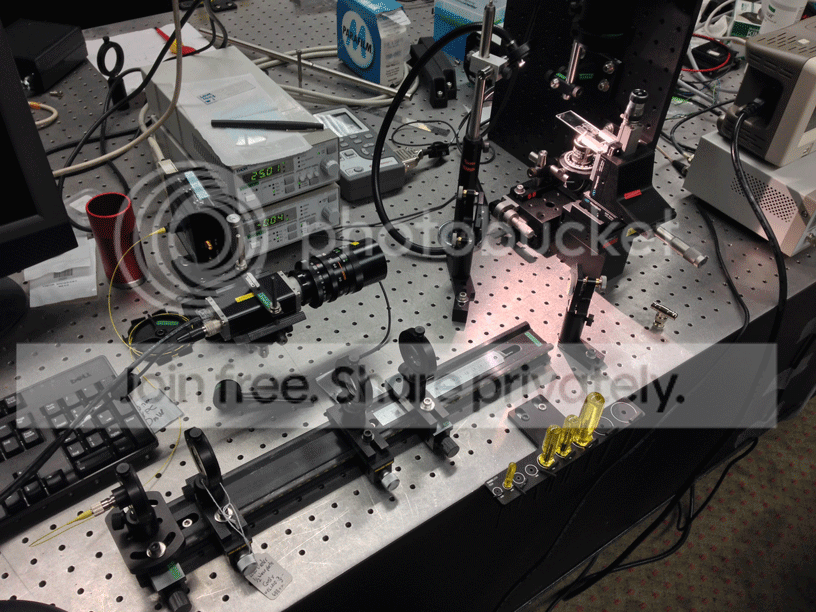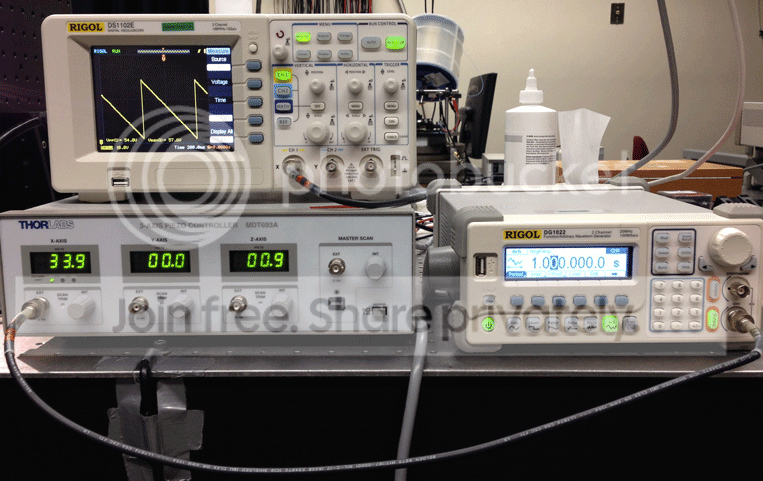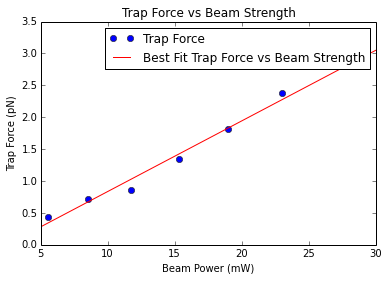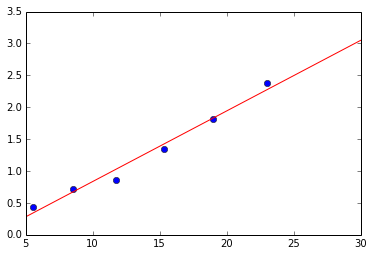# Stokes' Drag Force and Trap Forces¶

#### Measuring trap forces using stokes' drag force</br> </br> Brian Perrett, Samuel Estrella, Kyle Eichenberger</br> 5/28/14</br> Advanced Optics Lab 491</br> University of Oregon¶

$F_d = 6 \pi \mu R v_s$ </br> </br> $\mu$ is the dynamic viscosity $(8.90*10^{-4}Pa*s)$ </br> $R$ is the radius of the sphere </br> $v_s$ is the particle's velocity

We slowly accelerate the particle on a translational stage until the sphere breaks from the trap. We measure the velocity at which the sphere breaks from the trap to calculate our drag force, and equate it to the trap stiffness

$F_d = k$ </br>where $k$ is our trap force

##### Devices and Setup## RESULTS¶

Stoke's Drag Force Measurements
Beam Power (mW) Escape Velocity (microns/second) Trap Force (pN)
5.5 20.57 0.44
8.5 33.49 0.72
11.7 40.00 0.86
15.3 62.60 1.34
19.0 84.71 1.82
23.0 110.77 2.38
In :
plot(beam_strengths, trap_strengths, 'bo', label='Trap Force')
plot(line, fit, 'r-', label='Best Fit Trap Force vs Beam Strength')
title('Trap Force vs Beam Strength')
xlabel('Beam Power (mW)')
ylabel('Trap Force (pN)')
legend()
print("Slope is " + str(p))

Slope is 0.110650667695# Calculations¶

In :
mu = 8.9e-4 #Pa*S
R = 2.56e-6/2 #meters


## 5.5mW Beam Strength </br> T = 0.7 seconds Vpp = 54V

In :
T = .7 #seconds
V = 54 #Volts
dist = (20e-6*54)/75
v_s = dist/T
print("v_s is " + str(v_s))
F_d = 6*pi*mu*R*v_s
print("F_d is " + str(F_d))
k1 = F_d

v_s is 2.0571428571428573e-05
F_d is 4.417388044625202e-13


## 8.5mW Beam Strength </br> T = 0.43 seconds Vpp = 54V

In :
T = .43 #seconds
V = 54 #Volts
dist = (20e-6*54)/75
v_s = dist/T
print("v_s is " + str(v_s))
F_d = 6*pi*mu*R*v_s
print("F_d is " + str(F_d))
k2 = F_d

v_s is 3.348837209302325e-05
F_d is 7.191096816831723e-13


## 11.7mW Beam Strength </br> T = 0.36 seconds Vpp = 54V

In :
T = .36 #seconds
V = 54 #Volts
dist = (20e-6*54)/75
v_s = dist/T
print("v_s is " + str(v_s))
F_d = 6*pi*mu*R*v_s
print("F_d is " + str(F_d))
k3 = F_d

v_s is 3.9999999999999996e-05
F_d is 8.58936564232678e-13


## 15.3mW Beam Strength </br> T = 0.23 seconds Vpp = 54V

In :
T = .23 #seconds
V = 54 #Volts
dist = (20e-6*54)/75
v_s = dist/T
print("v_s is " + str(v_s))
F_d = 6*pi*mu*R*v_s
print("F_d is " + str(F_d))
k4 = F_d

v_s is 6.260869565217391e-05
F_d is 1.3444224483641918e-12


## 19.0mW Beam Strength </br> T = 0.17 seconds Vpp = 54V

In :
T = .17 #seconds
V = 54 #Volts
dist = (20e-6*54)/75
v_s = dist/T
print("v_s is " + str(v_s))
F_d = 6*pi*mu*R*v_s
print("F_d is " + str(F_d))
k5 = F_d

v_s is 8.470588235294117e-05
F_d is 1.8189244889633183e-12


## 23.0mW Beam Strength </br> T = 0.13 seconds Vpp = 54V

In :
T = .13 #seconds
V = 54 #Volts
dist = (20e-6*54)/75
v_s = dist/T
print("v_s is " + str(v_s))
F_d = 6*pi*mu*R*v_s
print("F_d is " + str(F_d))
k6 = F_d

v_s is 0.00011076923076923076
F_d is 2.378593562490493e-12

In :
beam_strengths = [5.5, 8.5, 11.7, 15.3, 19.0, 23.0] #mW
trap_strengths = array([k1, k2, k3, k4, k5, k6]) #N
trap_strengths = trap_strengths*1e12 #convert to pN

In :
plot(beam_strengths,trap_strengths, 'bo')
p = polyfit(beam_strengths, trap_strengths, 1)
line = linspace(5,30,2)
fit = p + p*line
plot(line, fit, 'r-')

Out:
[<matplotlib.lines.Line2D at 0x8d2b198>]These calculations turned out better than I had imagined they would. There is bit of uncertainty in deciding exactly when the spheres escape the optical trap, but it worked out quite well here.

Using this data, we can extrapolate what our Trap Force would be at the maximum intensity of 67.8mW.

In :
p + p*67.8

Out:
7.2317352916171069

We would expect a trap force of 7.23pN at an intensity of 67.8mW